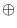Next: Example for a Stream-Cipher Up: Symmetric Key Cryptography Previous: Key for Cryptography (   Contents   Index

# Encryption and Decryption with Ciphers

A stream-cipher is a coder that encrypts or decrypts streams of data. An example for such a coder is the class CBCCoder that we have already seen in the sample program at the beginning of this chapter. Every stream-cipher in LEDA uses a block-cipher as building block. A block-cipher operates on ``small'' fixed-size blocks of plaintext or ciphertext - usually 64 or 128 bits. Mathematically, a block-cipher can be seen as pair of two functions EK and DK that depend on a key K. EK takes as input a block B of size b and returns the encrypted block (also of size b), DK describes the decryption operation. We have DK(EK(B)) = B for all blocks B of size b and every admissible key K.

A stream-cipher is usually built by combining a block-cipher and some sort of feedback via some simple operations. The most simple stream-cipher is the electronic codebook (ECB) mode: No chaining is used. The plaintext is partitioned into n blocks P1,..., Pn of size b. (If the last block Pn is shorter than b it is padded appropriately.) The ciphertext blocks C1,..., Cn are obtained by applying the block-cipher to each block of the plaintext. More formally, Ci = EK(Pi). Decryption is simple, too: Pi = DK(Ci). This simplicity has an important drawback: If a block B appears several times in the plaintext each occurence encrypts to the same ciphertext block. This can be exploited by an attacker: Suppose that the attacker knows P1 (maybe because every plaintext starts with a fixed header) then he is able to detect every occurence of P1 in the plaintext without knowing the key!

To overcome this serious drawback stream-ciphers with feedback have been invented. They hide patterns in the plaintext in the ciphertext. In cipher block chaining (CBC) mode the current plaintext block is XORed with the previous ciphertext block (as feedback) before encryption. In short, Ci = EK(PiCi-1). And decryption works as follows: Pi = DK(Ci)Ci-1. In order to compute C1 we need a so-called initialization vector (IV) C0. The IV does not have to be secret but it should be unique for every plaintext stream that is encrypted with a particular key K. The reason is the following: If you encrypt two streams with the same key and the same IV they will encrypt to same ciphertext up to the first position where the two plaintexts differ.

Another feedback mode is the cipher feedback (CFB) mode. It also uses an initialization vector C0. Encryption and decryption work as follows: Ci = PiEK(Ci-1) and Pi = CiEK(Ci-1). (Observe that the block-cipher is only used in encryption mode.) The IV for CFB must be unique (for CBC it should be unique), otherwise a cryptoanalyst can recover the plaintext (see [80, Chapter 9.6]).

Finally, we want to describe the output feedback (OFB) mode. It computes a feedback stream S0, S1,..., Sn, where S0 is an IV that should be unique. Despite the name of this mode, the feedback stream is completely internal it depends neither on the plaintext nor on the ciphertext: Si = EK(Si-1). This mode looks as follows: Ci = PiSi and Pi = CiSi.

Let us compare the four stream-ciphers from above. In terms of efficiency they are almost identical. The number of ciphertext blocks equals the number of plaintext blocks (not counting the IV). ECB is the fastest mode but the total running time is dominated by the time consumed by the block-cipher, the XOR operations used in the other modes are negligible. With respect to fault tolerance OFB is the best: One bit error in the ciphertext affects only one bit in the decrypted plaintext. In ECB mode one bit in the ciphertext affects the complete corresponding plaintext block. For CBC it affects the full corresponding block in the plaintext and one bit in the next block. And in CFB mode the corresponding bit in the plaintext is affected as well as the complete next block. As to security ECB is clearly the worst because patterns in the plaintext are not hidden. The other three modes hide these patterns and they are almost comparable in terms of security. CBC has some small advantages (cf. [80, Chapter 9.11]). So if your are in doubt use CBC as stream-cipher.
The following table summarizes some facts about the stream-ciphers in LEDA:

 mode encryption decryption IV security ECB Ci = EK(Pi) Pi = DK(Ci) none - CBC Ci = EK(PiCi-1) Pi = DK(Ci)Ci-1 unique + (+) CFB Ci = PiEK(Ci-1) Pi = CiEK(Ci-1) unique! + OFB Ci = PiSi, Si = EK(Si-1) Pi = CiSi unique +

As stated above a block-cipher is needed to make the stream-ciphers work. The following block-ciphers are part of LEDA:

 block-cipher block size / bits key-size / bits Blowfish 64 32 - 448 Twofish 128 128 - 256 Rijndael (AES) 128 128 - 256

Comparing the ciphers in terms of performance Blowfish comes out worst (probably because of the smaller block size). Our implementation of Twofish is slightly faster than that of Rijndael. In terms of security it is hard to rank the three ciphers. All of them have been intensively cryptoanalyzed and no weaknesses have been found so far. Blowfish is used in the ssh command of Unix, Rijndael won the contest for the Advanced Encryption Standard (AES), and Twofish reached the final round of that contest.Next: Example for a Stream-Cipher Up: Symmetric Key Cryptography Previous: Key for Cryptography (   Contents   Index